# Recursion You will learn the definition of recursion

• Slides: 36Recursion You will learn the definition of recursion as well as seeing how simple recursive programs workWhat Is Recursion? “the determination of a succession of elements by operation on one or more preceding elements according to a rule or formula involving a finite number of steps” (Merriam-Webster online)What This Really Means Breaking a problem down into a series of steps. The final step is reached when some basic condition is satisfied. The solution for each step is used to solve the previous step. The solution for all the steps together form the solution to the whole problem. (The “Tam” translation)Definition Of Philosophy “…state of mind of the wise man; practical wisdom…” 1 See Metaphysics 1 The New Webster Encyclopedic Dictionary of the English Language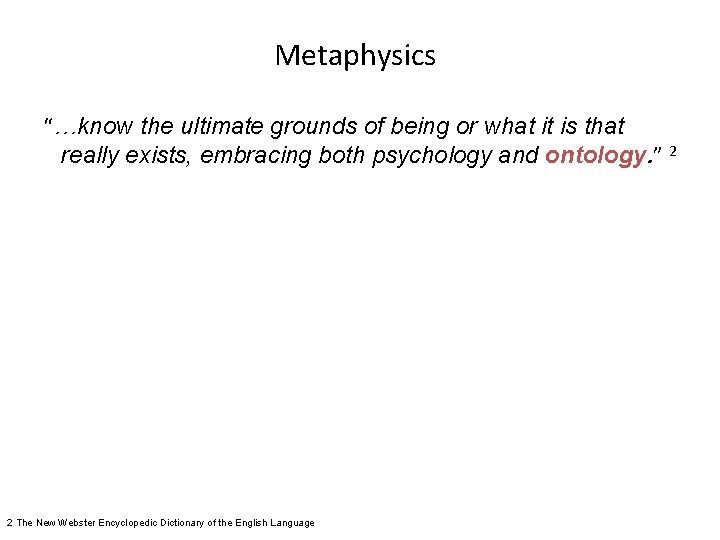Metaphysics “…know the ultimate grounds of being or what it is that really exists, embracing both psychology and ontology. ” 2 2 The New Webster Encyclopedic Dictionary of the English Language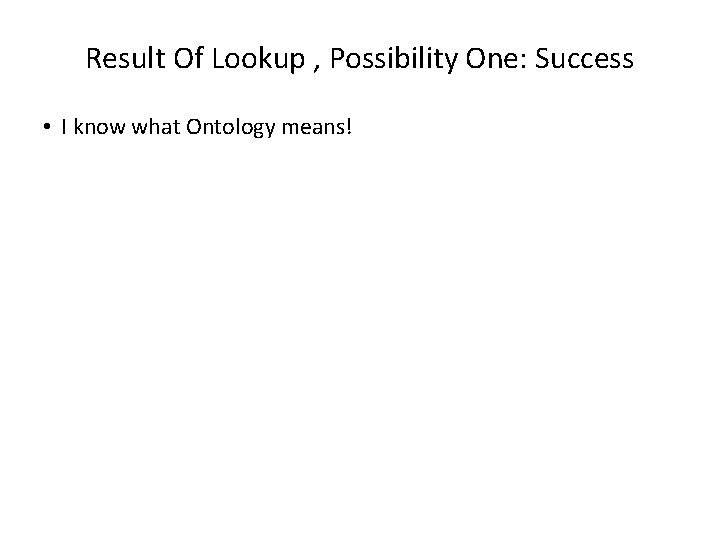Result Of Lookup , Possibility One: Success • I know what Ontology means!Result Of Lookup, Possibility One Philosophy? Metaphysics? Success! I’ll take a Philosophy option. Ontology!Result Of Lookup, Possibility Two: Failure • Lookup ‘loops’ back.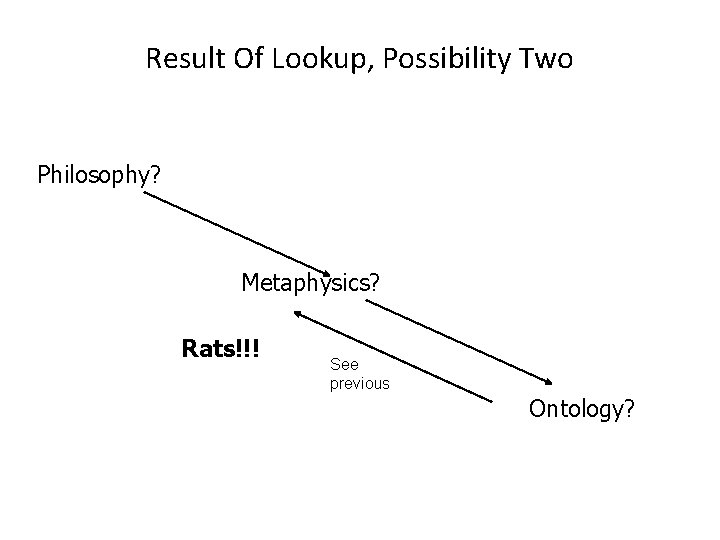Result Of Lookup, Possibility Two Philosophy? Metaphysics? Rats!!! See previous Ontology?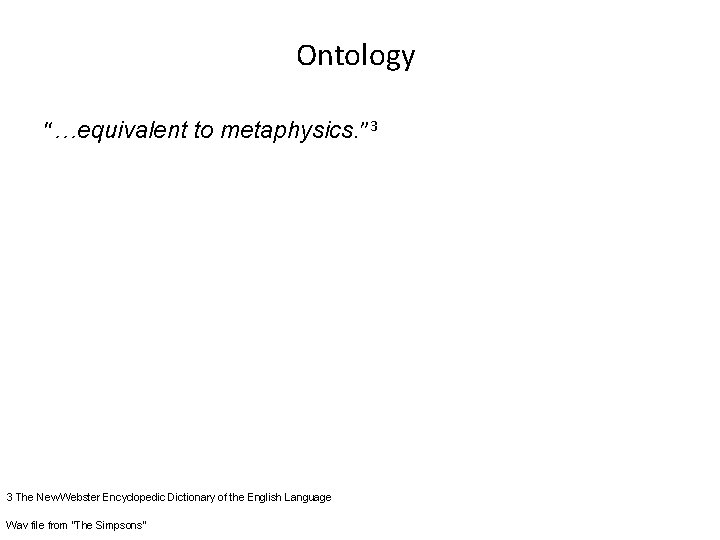Ontology “…equivalent to metaphysics. ” 3 3 The New Webster Encyclopedic Dictionary of the English Language Wav file from “The Simpsons”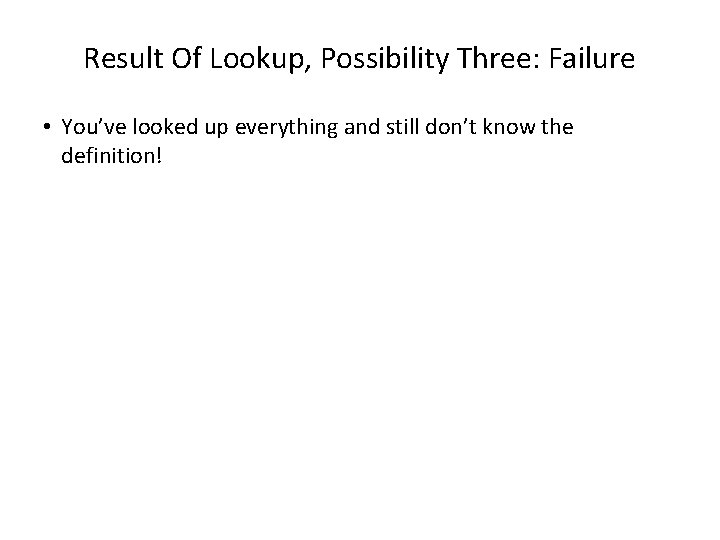Result Of Lookup, Possibility Three: Failure • You’ve looked up everything and still don’t know the definition!Looking Up A Word if (you completely understand a definition) then return to previous definition (using the definition that’s understood) else lookup (unknown word(s))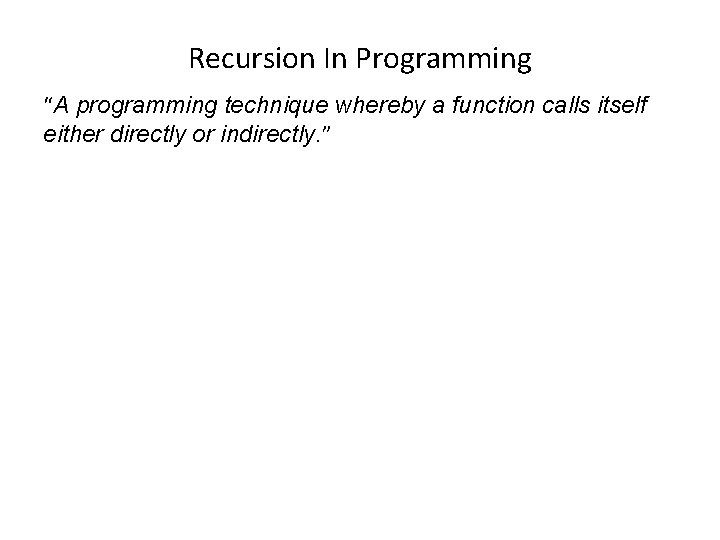Recursion In Programming “A programming technique whereby a function calls itself either directly or indirectly. ”Direct Call def fun (): … function fun () …Indirect Call f 1 f 2Indirect Call f 1 f 2 f 3 … fn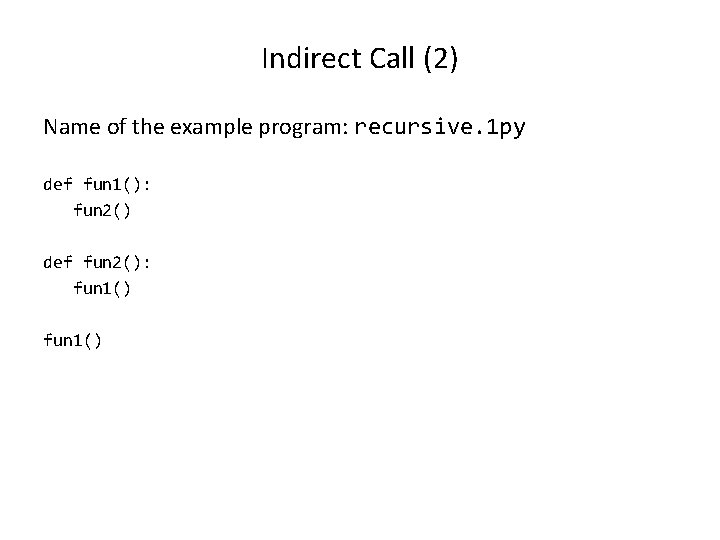Indirect Call (2) Name of the example program: recursive. 1 py def fun 1(): fun 2() def fun 2(): fun 1()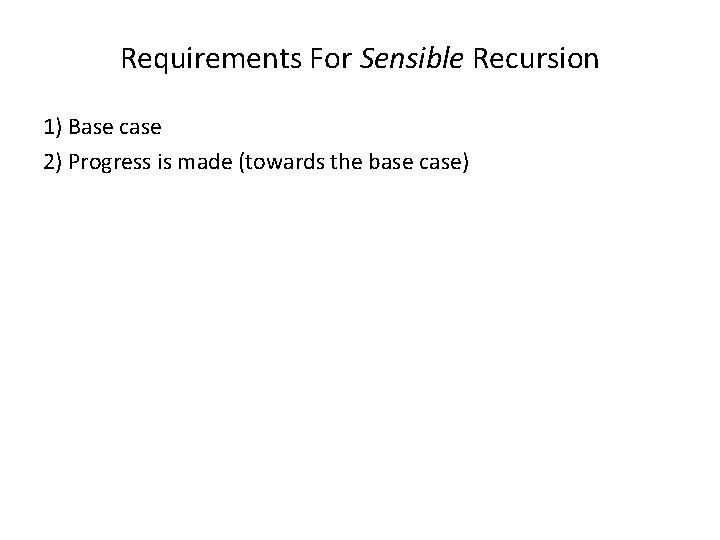Requirements For Sensible Recursion 1) Base case 2) Progress is made (towards the base case)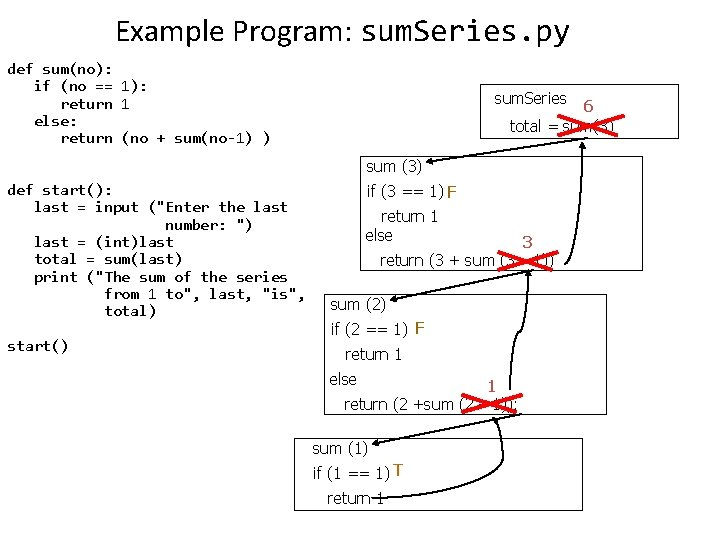Example Program: sum. Series. py def sum(no): if (no == 1): return 1 else: return (no + sum(no-1) ) sum. Series 6 total = sum(3) sum (3) def start(): last = input ("Enter the last number: ") last = (int)last total = sum(last) print ("The sum of the series from 1 to", last, "is", total) start() if (3 == 1) F return 1 else 3 return (3 + sum (3 – 1)) sum (2) if (2 == 1) F return 1 else 1 return (2 +sum (2 – 1)); sum (1) if (1 == 1) T return 1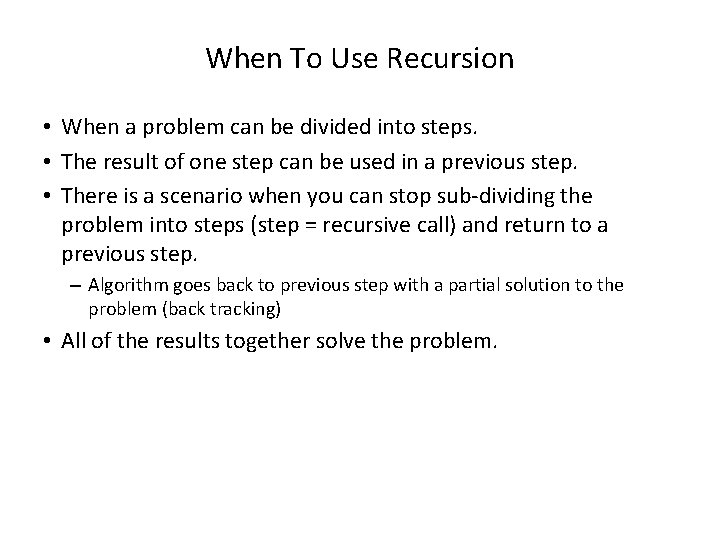When To Use Recursion • When a problem can be divided into steps. • The result of one step can be used in a previous step. • There is a scenario when you can stop sub-dividing the problem into steps (step = recursive call) and return to a previous step. – Algorithm goes back to previous step with a partial solution to the problem (back tracking) • All of the results together solve the problem.When To Consider Alternatives To Recursion • When a loop will solve the problem just as well • Types of recursion (for both types a return statement is excepted) – Tail recursion • The last statement in the function is another recursive call to that function This form of recursion can easily be replaced with a loop. – Non-tail recursion • The last statement in the recursive function is not a recursive call. • This form of recursion is very difficult (read: impossible) to replace with a loop.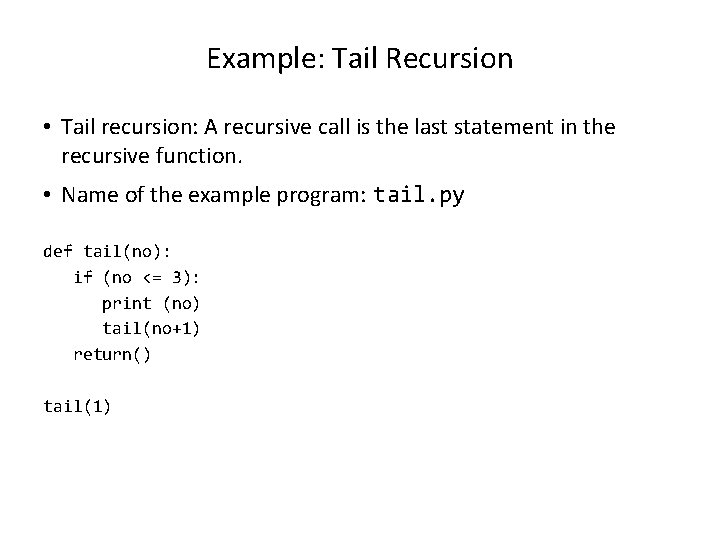Example: Tail Recursion • Tail recursion: A recursive call is the last statement in the recursive function. • Name of the example program: tail. py def tail(no): if (no <= 3): print (no) tail(no+1) return() tail(1)Example: Non-Tail Recursion • Non-Tail recursion: A statement which is not a recursive call to the function comprises the last statement in the recursive function. • Name of the example program: non. Tail. py def non. Tail(no): s if (no < 3): non. Tail(no+1) print(no) return() non. Tail(1)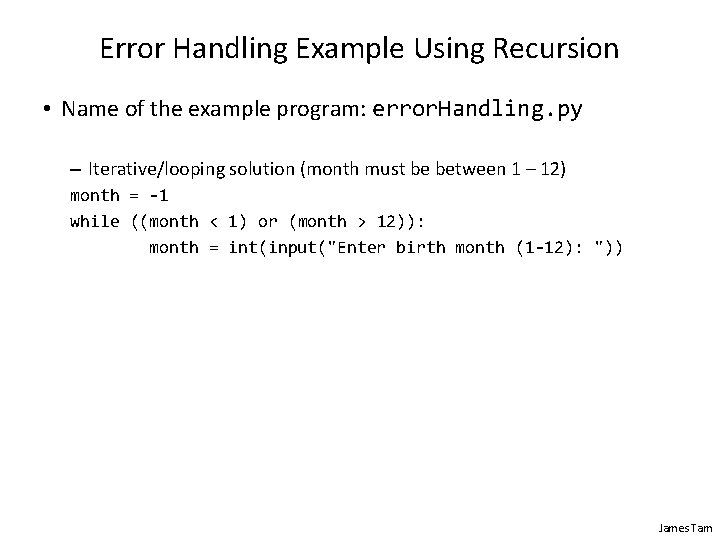Error Handling Example Using Recursion • Name of the example program: error. Handling. py – Iterative/looping solution (month must be between 1 – 12) month = -1 while ((month < 1) or (month > 12)): month = int(input("Enter birth month (1 -12): ")) James Tam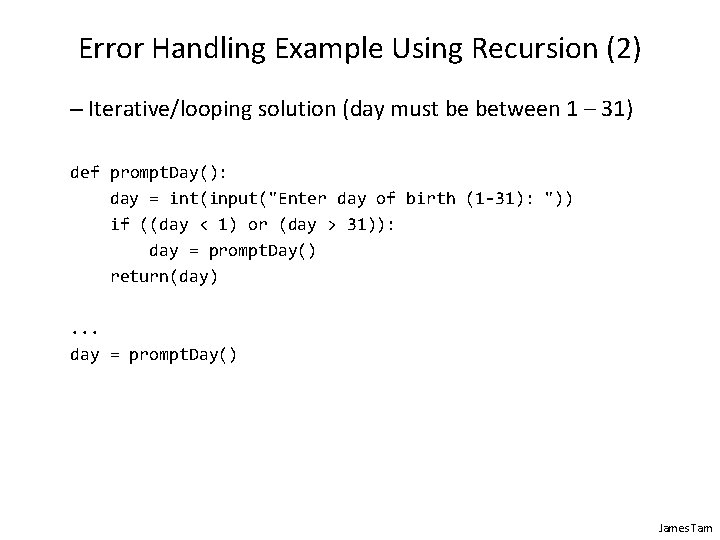Error Handling Example Using Recursion (2) – Iterative/looping solution (day must be between 1 – 31) def prompt. Day(): day = int(input("Enter day of birth (1 -31): ")) if ((day < 1) or (day > 31)): day = prompt. Day() return(day). . . day = prompt. Day() James Tam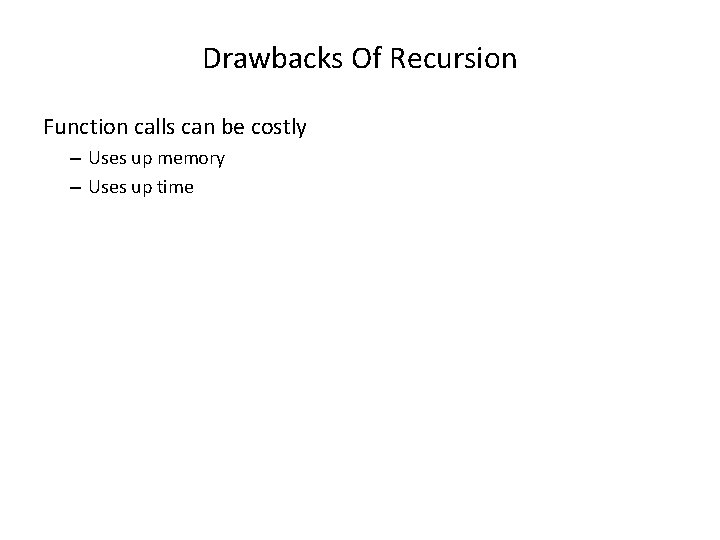Drawbacks Of Recursion Function calls can be costly – Uses up memory – Uses up time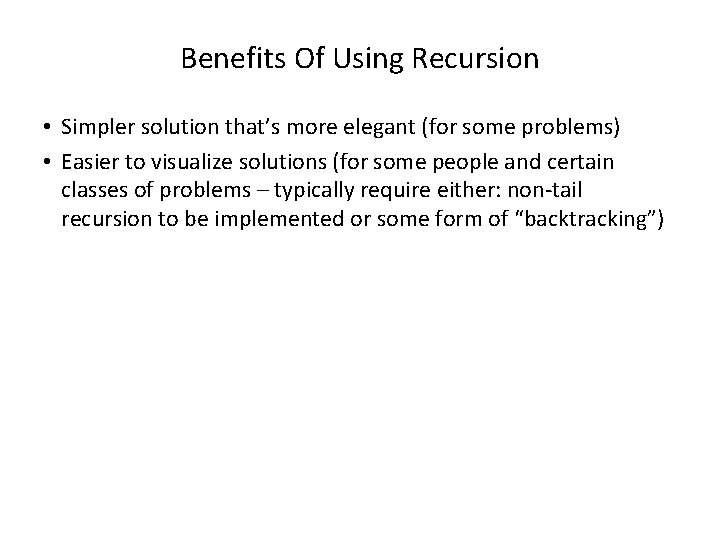Benefits Of Using Recursion • Simpler solution that’s more elegant (for some problems) • Easier to visualize solutions (for some people and certain classes of problems – typically require either: non-tail recursion to be implemented or some form of “backtracking”)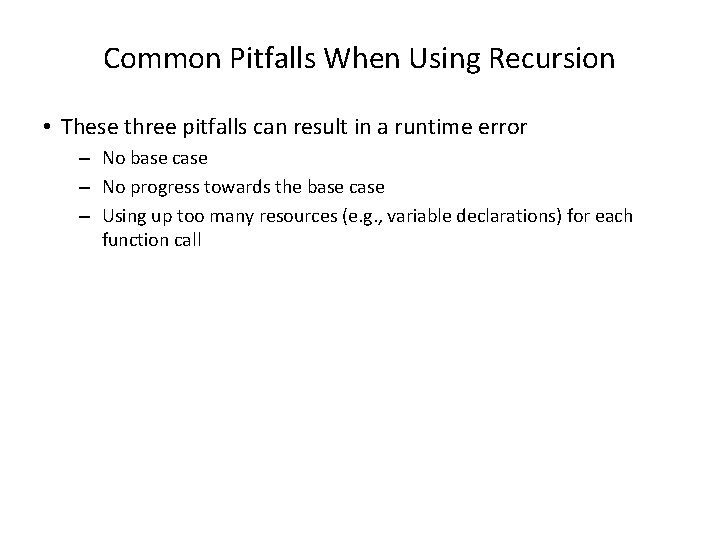Common Pitfalls When Using Recursion • These three pitfalls can result in a runtime error – No base case – No progress towards the base case – Using up too many resources (e. g. , variable declarations) for each function callNo Base Case def sum(no): return(no + sum (no - 1))No Base Case def sum (no): return (no + sum (no - 1)) When does it stop? ? ?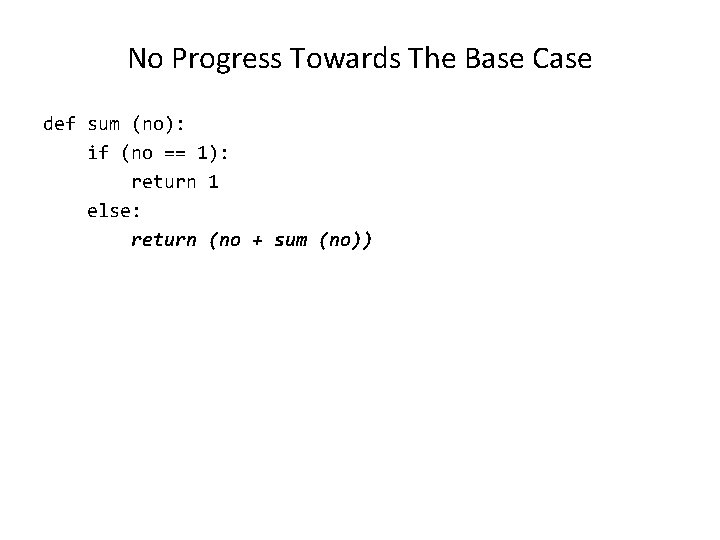No Progress Towards The Base Case def sum (no): if (no == 1): return 1 else: return (no + sum (no))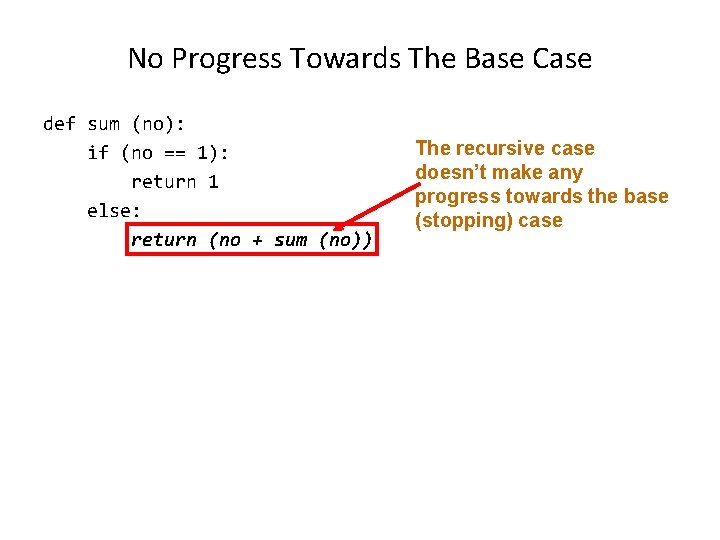No Progress Towards The Base Case def sum (no): if (no == 1): return 1 else: return (no + sum (no)) The recursive case doesn’t make any progress towards the base (stopping) case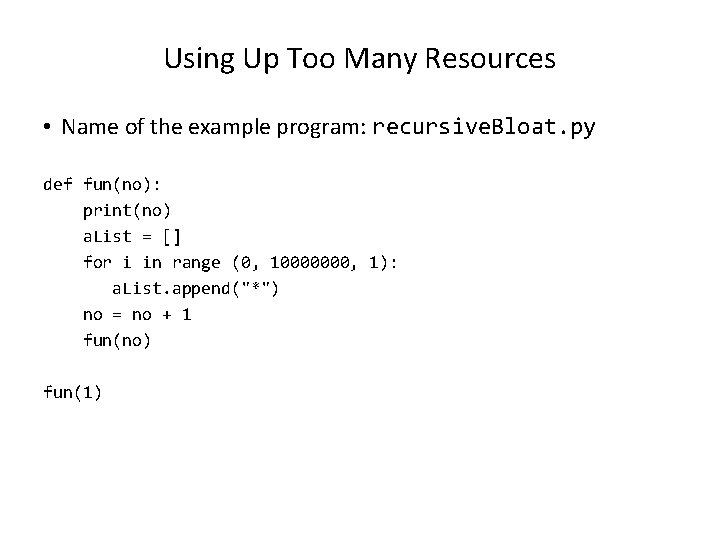Using Up Too Many Resources • Name of the example program: recursive. Bloat. py def fun(no): print(no) a. List = [] for i in range (0, 10000000, 1): a. List. append("*") no = no + 1 fun(no) fun(1)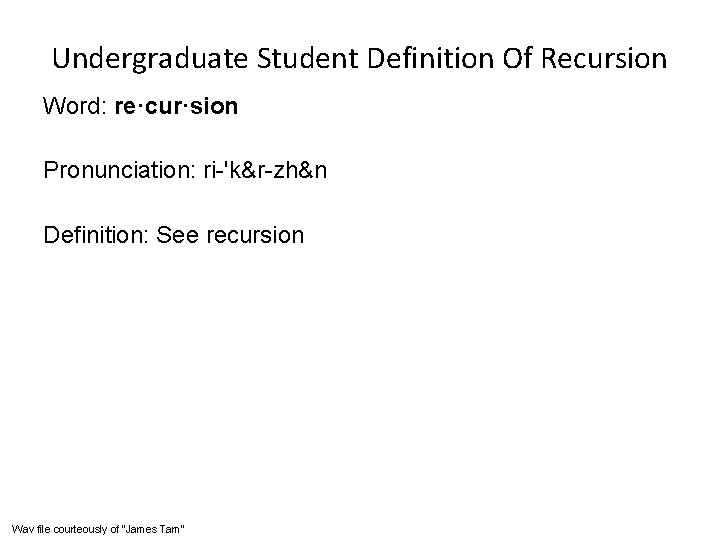Undergraduate Student Definition Of Recursion Word: re·cur·sion Pronunciation: ri-'k&r-zh&n Definition: See recursion Wav file courteously of “James Tam”Recursion: Job Interview Question • http: //www. businessinsider. com/apple-interview-questions-2011 -5#write-a-function-that-calculates-a-numbers-factorialusing-recursion-9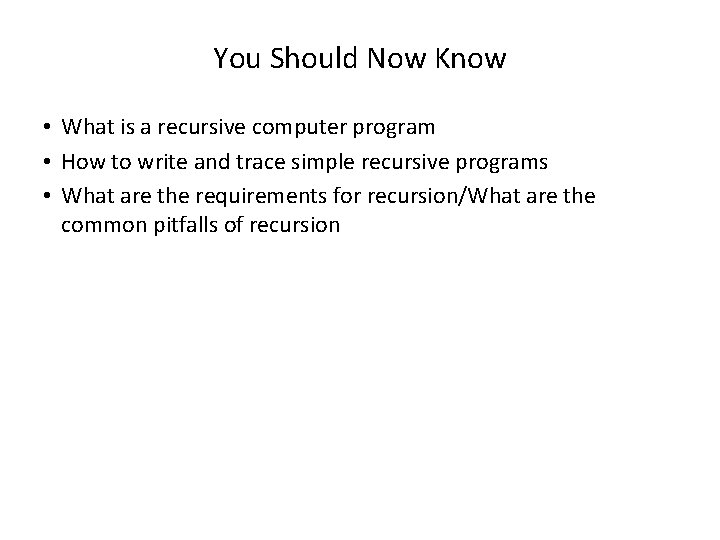You Should Now Know • What is a recursive computer program • How to write and trace simple recursive programs • What are the requirements for recursion/What are the common pitfalls of recursion